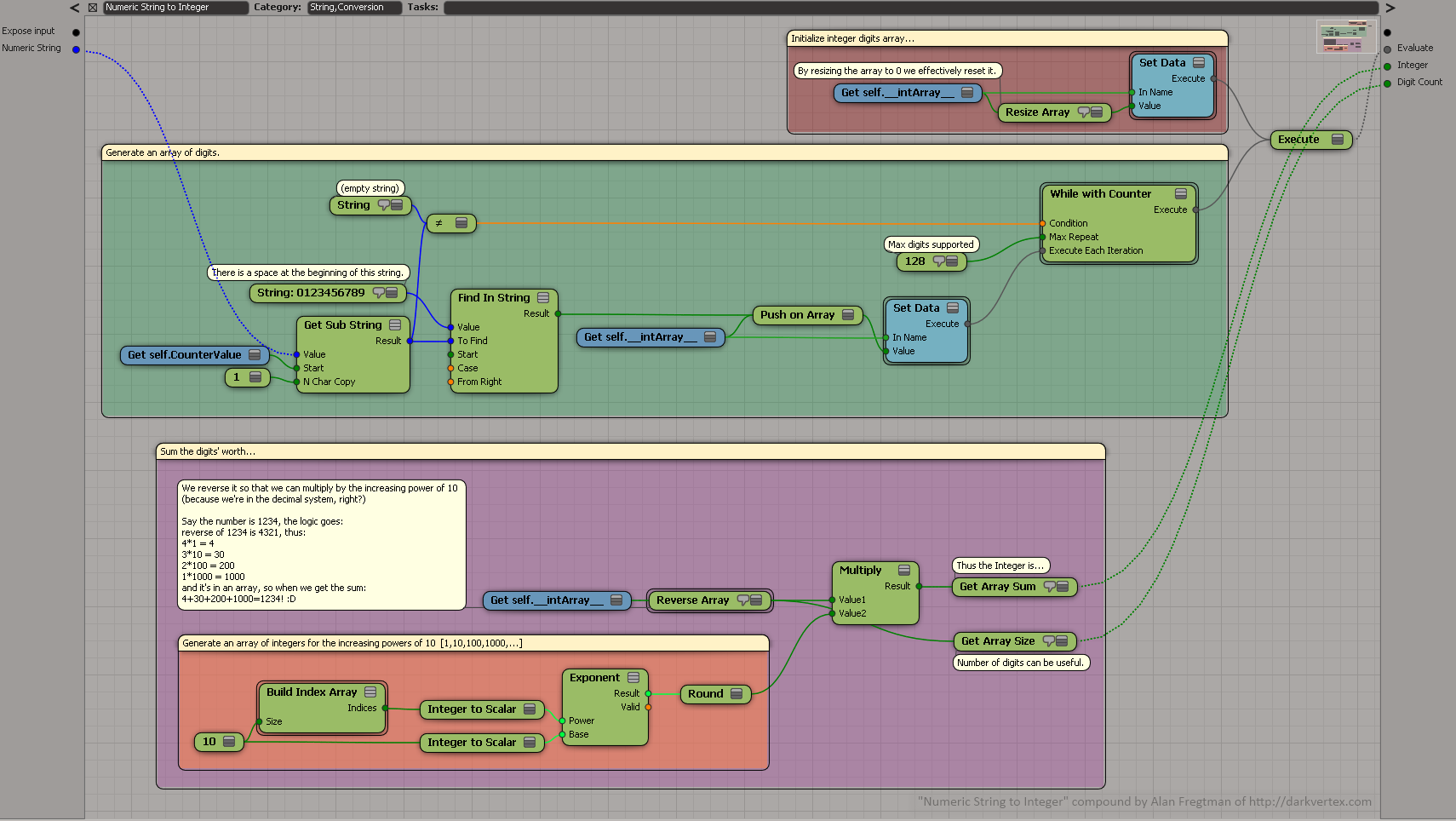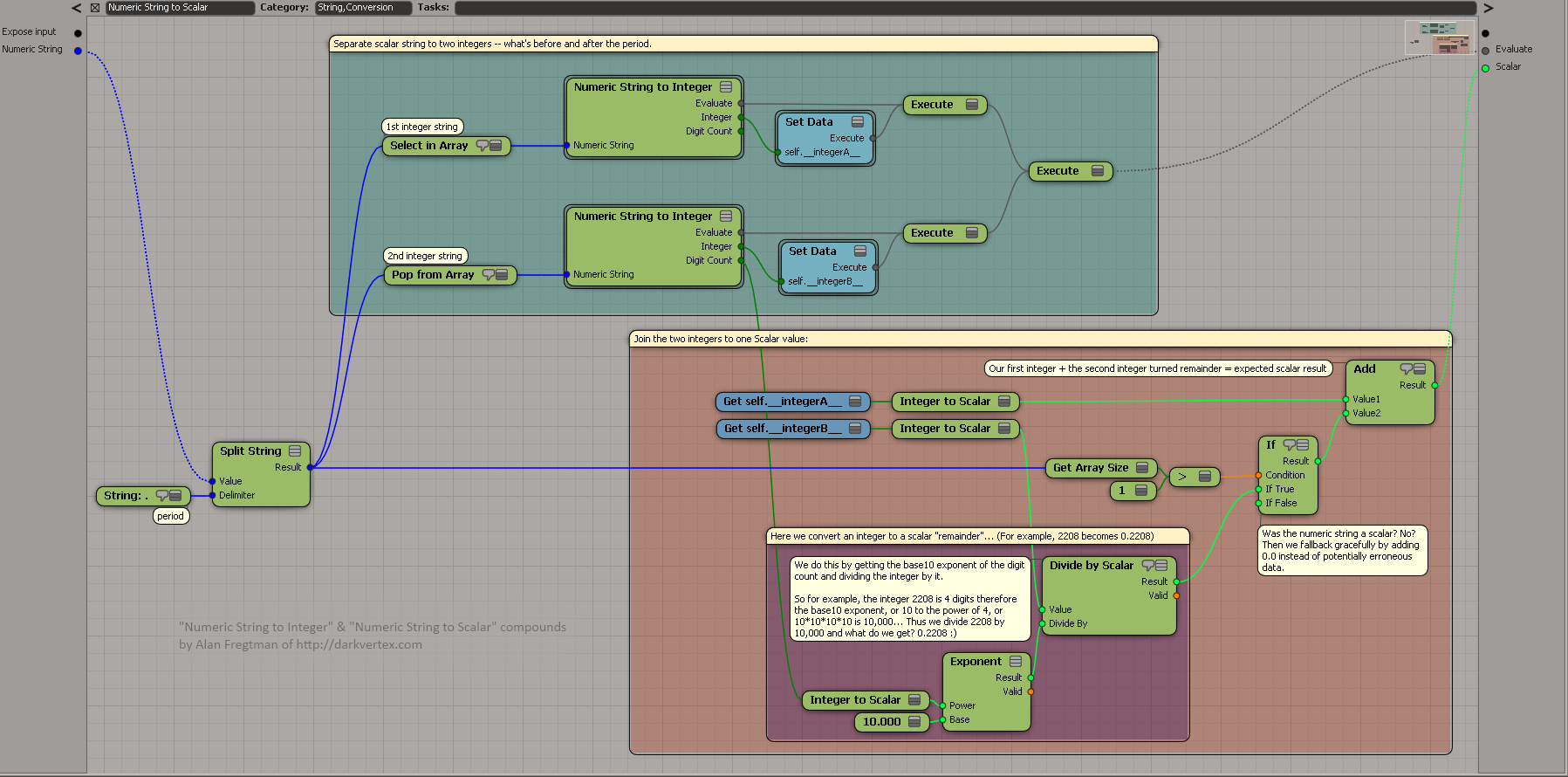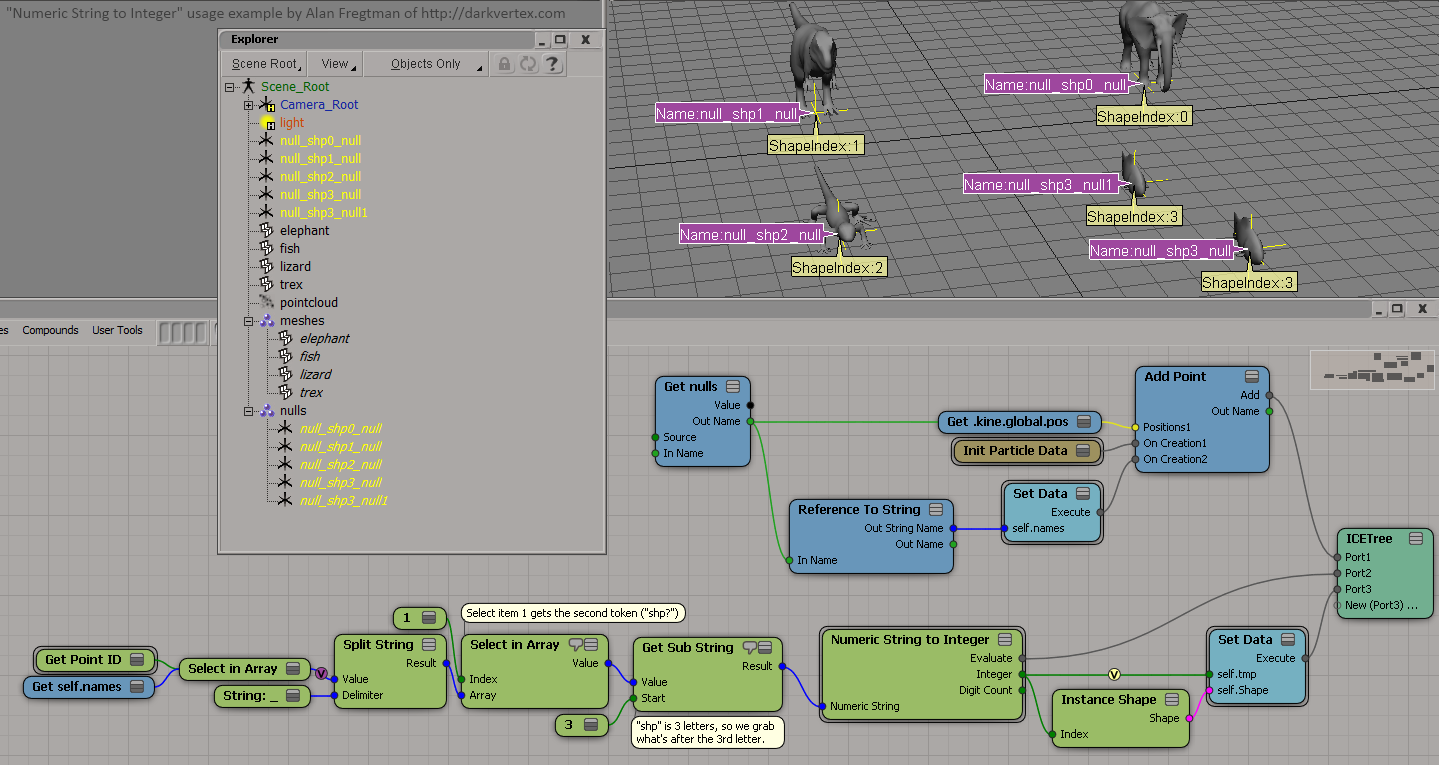2012
08.15

## ICE Strings Revisited: Numeric String to Integer or Scalar

Some of you may remember I wrote about some ICE trickery with strings some years ago. The other day I pondered if one could turn a string of digits into an integer or scalar (float) value. Well, I’ve found a way!

# String to Integer – ICEtree:Basically you loop digit by digit and find the index in the string “012345679” that your digit corresponds, thus giving you each single digit as an integer. We proceed to combine the digits to an array. When done, that digit array is reversed and each single digit is multiplied by the corresponding powers of 10, thus giving us what the digit was worth. The array sum of these calculations becomes the integer we’re after.

For example, let’s say we have the number “1234” or “one thousand two hundred and four“:
Finding each digit in the string “1234” we get the array [1,2,3,4]
reversed it is of course [4,3,2,1]
so we iterate digit by digit, multiplying by increasing exponentials of ten like so:
4 * 1 = 4
3 * 10 = 30
2 * 100 = 200
1 * 1000 = 1000
so now we have an array of integers [4,30,200,1000]
so we sum it up and what do we get?
4+30+200+1000=1234 as an integer value!

# String to Scalar – ICEtree:To do an ICE String to a Scalar (a number with a decimal value, a.k.a. a “float”) we can fundamentally do the above algorithm twice, by treating the integer and the remainder (what’s after the period) as individual integer values.

For example say we have a scalar string saying “1234.567”, we split by the period (.) and interpret the strings “1234” and “567” individually with our integer conversion algorithm. However, when adding the remainder (567) we can’t add it directly as that would be 1234+567=1801 which is NOT what we want! The trick is to count the digits:
567 is 3 digits,
and 10 to the 3rd power, or 10*10*10 is 1000,
therefore we can divide 567 by 1000 and we get 0.567,
so then we can do 1234 + 0.567 = 1234.567, to get the Scalar we want! 😀

(Remember though, that you may get a slightly different remainder due to floating point inaccuracies when interpreting the number.)

# FUN USAGE EXAMPLES 😀

Reference to String” is a fantastic node. When fed a GetData’s OutName it will give you a string, but the best is if you feed it a magic keyword (this, self, this_model, this_parent) it resolves the real object name AND even better, check this:^ If you feed it a group, you get an array of strings of the groups’ member names. Cool, huh? 🙂

This means we can encode settings into numbers of the object names and read them from ICE. Here’s an example where the object name decides the shape index:Now here’s a different example where I take the number at the end of object’s name to decide the index to constrain to:So tell me, what will YOU do with ICE strings now?

[NOTE:
There seems to currently be a minor refresh bug with the “Reference to String” node. When renaming objects it doesn’t refresh so if you want your tree to update you may have to reconnect the input to the “Reference to String” node OR reopen your scene; that works too.]

1.Thanks.

2.and post it to the list when you do them from now on, screw the reg’s 🙂

Thanks 2